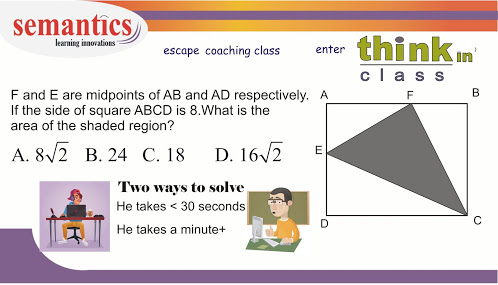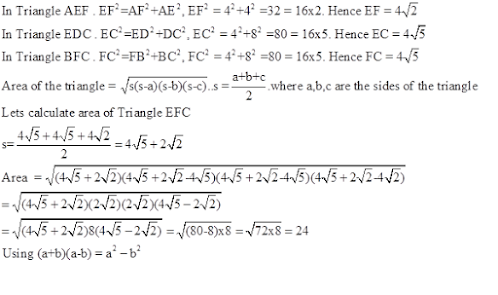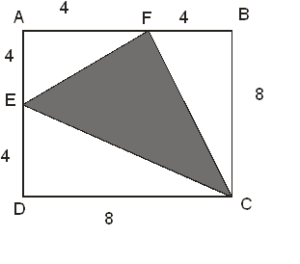# math problem solving skills 1

To ace the math section, you need to remember all the relevant math concepts as well as develop problem-solving skills.

Today I am going to take you through one problem-solving skill –

## Diagramming

Lets take this problem .I will take you through two approaches.

You will use many formulas and theorems. You will get an answer, but it will take more time.

You will minimize the number of formulas used. You will use your logical skills, hence reduce complex computation. You will solve  faster.

If you solve questions in less than 30 seconds, then you will have more time in the bank to solve harder questions. You will also be able to complete the section in the allotted time.

Penalty marks for un-attempted questions are huge.

Can logic be taught?

Yes!  Logic can be taught. If the tutor teaches you reasoning skills and demonstrates those skills on a wide range of problems, your thinking will get re-oriented. You will be able to solve questions using more than one approach.

I feel logic is best taught in a tutor driven class, not through generic videos

Now let us understand both the approaches.

## Approach 1

This approach involves formulae/theorem…Area of square ABCD = side2

Side = 8. Hence area =64

F and E are midpoints of the respective sides. AB=AD=8

Hence AF=FB=4 and AE=ED=4

Triangle AEF, Triangle BFC and Triangle EDC are right angled triangles. Hence we can use Pythagoras theoremThis approach is time consuming. Also, this approach involves lots of calculation.

## Approach 2 – A faster approachWhen you encounter geometry problems, look at the picture for few secondsCan you observe a square and 4 triangles?

Spend time observing the pictures and look for clues.

The area of shaded portion is equal to the area of the square – (sum of the area of the 3 triangles).

This approach requires you to know the area of the triangle = 0.5 x base x height.

Now let’s analyze the figure. F and E are the midpoints

The sides of the square are 8

Area of triangle AEF = 0.5 x 4 x 4 = 8

Area of triangle EDC = 0.5 x 4 x 8 = 16

Area of triangle FBC = 0.5 x 8 x 4 = 16

Area of square =64

Area of shaded region = 64- 16-16-8 =24

This approach is far easier and involves less calculation.

So always use logic to arrive at answers faster

Need a math refresher course.?

Need a math course, which teaches math logic?

Get in touch with us

Call Now Button